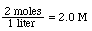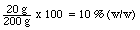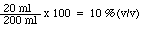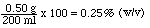Solution Calculations

## Solution Calculations

The concentration of a substance in a solution or mixture can be expressed in a number of ways. Four of the most common are defined below:

Molarity (M) = moles of solute / liters of solution
ex. 80.0 g of NaOH dissolved in enough water to make 1 liter of solution would result in a 2.00 M NaOH solutionPercent weight (%(w/w)) = 100 x (mass of solute / mass of solution)
ex. 20 g of NaCl added to enough sand to make 200 g of mixture would result in a 10 % (w/w) NaCl/sand mixture-the units may be any units of mass you chose - as long as they are consistent.
-this concentration unit is most often used when mixing two solids

Percent volume-volume (%(v/v)) = 100 x (volume of solute / volume of solution)
ex. 20 ml of methanol dissolved in enough water to make 200 ml of solution would result in a 10 % methanol solution-the units may be any units of volume you chose - as long as they are consistent
-this concentration unit is most often used when mixing two liquids

Percent weight-volume (%(w/v)) = 100 x (g of solute / ml of solution)
ex. 0.50 g of NaCl dissolved in enough water to make 200 ml of solution would result in a 0.25 % (w/v) methanol solution-the units must be g and ml
-this concentration unit is most often used when mixing a solid and a liquid